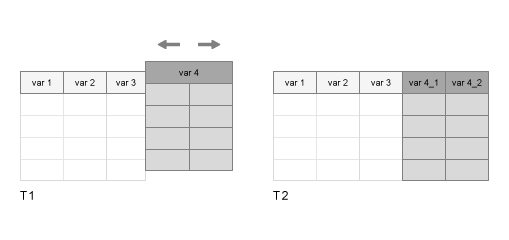# splitvars

Split multicolumn variables in table or timetable

## Syntax

``T2 = splitvars(T1)``
``T2 = splitvars(T1,vars)``
``T2 = splitvars(T1,vars,'NewVariableNames',newNames)``

## Description

example

````T2 = splitvars(T1)` splits all multicolumn variables in `T1` so that they are single-column variables in `T2` (see diagram). All single-column variables from `T1` are unaltered.If a variable in `T1` has multiple columns, then `splitvars` makes unique names for the new variables in `T2` from the name of the original variable in `T1`.If a variable in `T1` is a table itself, then `splitvars` uses the names of its variables (and, if necessary, the name of that table) to make unique names for the new variables in `T2`.To merge variables into one multicolumn variable, use the `mergevars` function.```

example

````T2 = splitvars(T1,vars)` splits only the table variables specified by `vars`. You can specify variables by name, by position, or using logical indices.```

example

````T2 = splitvars(T1,vars,'NewVariableNames',newNames)` assigns new names to the designated variables that are split out of `T1` and copied to `T2`.```

## Examples

collapse all

Create a table from workspace variables. Some of the variables are matrices with multiple columns.

```A = (1:3)'; B = [5 11 12; 20 30 50; 0.1 3.4 5.9]'; C = {'a','XX';'b','YY';'c','ZZ'}; D = [128 256 512]'; T1 = table(A,B,C,D)```
```T1=3×4 table A B C D _ ________________ _______________ ___ 1 5 20 0.1 {'a'} {'XX'} 128 2 11 30 3.4 {'b'} {'YY'} 256 3 12 50 5.9 {'c'} {'ZZ'} 512 ```

Split the variables `B` and `C`. All variables in the output table have one column.

`T2 = splitvars(T1)`
```T2=3×7 table A B_1 B_2 B_3 C_1 C_2 D _ ___ ___ ___ _____ ______ ___ 1 5 20 0.1 {'a'} {'XX'} 128 2 11 30 3.4 {'b'} {'YY'} 256 3 12 50 5.9 {'c'} {'ZZ'} 512 ```

Create a table that contains tables, using arrays of data from the `patients.mat` file. Display the first three rows.

```load patients Personal_Data = table(Gender,Age); BMI_Data = table(Height,Weight); BloodPressure = table(Systolic,Diastolic); T1 = table(LastName,Personal_Data,BMI_Data,BloodPressure); head(T1,3)```
```ans=3×4 table LastName Personal_Data BMI_Data BloodPressure Gender Age Height Weight Systolic Diastolic ____________ _________________ ________________ _____________________ {'Smith' } {'Male' } 38 71 176 124 93 {'Johnson' } {'Male' } 43 69 163 109 77 {'Williams'} {'Female'} 38 64 131 125 83 ```

Specify `BloodPressure` as the variable to split.

```T2 = splitvars(T1,'BloodPressure'); head(T2,3)```
```ans=3×5 table LastName Personal_Data BMI_Data Systolic Diastolic Gender Age Height Weight ____________ _________________ ________________ ________ _________ {'Smith' } {'Male' } 38 71 176 124 93 {'Johnson' } {'Male' } 43 69 163 109 77 {'Williams'} {'Female'} 38 64 131 125 83 ```

To specify multiple variables by name, use a cell array of character vectors.

```T3 = splitvars(T1,{'BMI_Data','BloodPressure'}); head(T3,3)```
```ans=3×6 table LastName Personal_Data Height Weight Systolic Diastolic Gender Age ____________ _________________ ______ ______ ________ _________ {'Smith' } {'Male' } 38 71 176 124 93 {'Johnson' } {'Male' } 43 69 163 109 77 {'Williams'} {'Female'} 38 64 131 125 83 ```

To specify variables by position, use a numeric array.

```T4 = splitvars(T1,[2 4]); head(T4,3)```
```ans=3×6 table LastName Gender Age BMI_Data Systolic Diastolic Height Weight ____________ __________ ___ ________________ ________ _________ {'Smith' } {'Male' } 38 71 176 124 93 {'Johnson' } {'Male' } 43 69 163 109 77 {'Williams'} {'Female'} 38 64 131 125 83 ```

Create a table that contains multi-column variables, using data from the `patients.mat` file. Display the first three rows.

```load patients Personal_Data = [Age,Height,Weight]; BloodPressure = [Systolic,Diastolic]; T1 = table(LastName,Gender,Personal_Data,BloodPressure); head(T1,3)```
```ans=3×4 table LastName Gender Personal_Data BloodPressure ____________ __________ ________________ _____________ {'Smith' } {'Male' } 38 71 176 124 93 {'Johnson' } {'Male' } 43 69 163 109 77 {'Williams'} {'Female'} 38 64 131 125 83 ```

Split `BloodPressure` and specify new names for the new variables in the output table.

```T2 = splitvars(T1,'BloodPressure','NewVariableNames',{'Systolic','Diastolic'}); head(T2,3)```
```ans=3×5 table LastName Gender Personal_Data Systolic Diastolic ____________ __________ ________________ ________ _________ {'Smith' } {'Male' } 38 71 176 124 93 {'Johnson' } {'Male' } 43 69 163 109 77 {'Williams'} {'Female'} 38 64 131 125 83 ```

Split both `BMI_Data` and `BloodPressure`. For each variable being split, you must provide a cell array with the correct number of new names.

```T3 = splitvars(T1,{'Personal_Data','BloodPressure'},... 'NewVariableNames',{{'Age','Height','Weight'},{'Systolic','Diastolic'}}); head(T3,3)```
```ans=3×7 table LastName Gender Age Height Weight Systolic Diastolic ____________ __________ ___ ______ ______ ________ _________ {'Smith' } {'Male' } 38 71 176 124 93 {'Johnson' } {'Male' } 43 69 163 109 77 {'Williams'} {'Female'} 38 64 131 125 83 ```

## Input Arguments

collapse all

Input table, specified as a table or timetable.

Variables in the input table, specified as a character vector, cell array of character vectors, string array, numeric array, or logical array.

Names of the split variables, specified as a cell array of character vectors or string array.

## Extended Capabilities

Introduced in R2018a